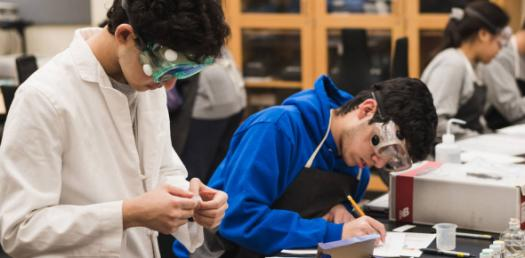# Can You Pass This Toughest Chemistry Exam? Trivia Quiz

50 Questions | Total Attempts: 39Settings.

• 1.
Atoms of the same element with different numbers of neutrons are called____.
• A.

Isotopes

• B.

Metals

• C.

Metalloids

• D.

• 2.
A certain atom has 38 protons, 38 electrons, and 50 neutrons. Its mass number is ____.
• A.

12

• B.

38

• C.

88

• D.

126

• 3.
Why are the nobles gases stable?
• A.

They were used by monarchies throughout history

• B.

They have a full number of outer electrons

• C.

They will not gain or lose electrons

• D.

Only b and c

• E.

All choices are correct

• 4.
An atom with a different number of electrons than protons is a(n) ____.
• A.

Isotope

• B.

Atom

• C.

Ion

• D.

Nucleon

• 5.
What periods and group, respectively, is barium found in?
• A.

6, 1

• B.

5, 2

• C.

2, 6

• D.

6, 2

• 6.
Given the following information 12853I, how many electrons are present?
• A.

75

• B.

128

• C.

53

• D.

127

• 7.
An atom is held together by the interactions of:
• A.

Protons and electrons

• B.

Protons and protons

• C.

Neutrons and electrons

• D.

All of the choices

• 8.
An atom that has gained two electrons would have what charge?
• A.

-2

• B.

+2

• C.

-6

• D.

Neutral

• 9.
Krypton is considered an inert atom. What makes Krypton such a stable atom?
• A.

8 electrons in the outer shell

• B.

More negative than positive charge

• C.

More positive than negative charge

• D.

None of the choices are correct

• 10.
Atoms found in period 4, group16 are considered to be ...
• A.

Metalloids

• B.

Metals

• C.

Non-metals

• D.

Mental

• 11.
In an atom, the atomic number is useful because a physical science student can determine the ...
• A.

Number of protons.

• B.

Number of quarks.

• C.

Atomic mass.

• D.

Ionic charge.

• 12.
Current uses of nuclear energy are beneficial to humankind except for the:
• A.

Carbon dioxide pollution.

• B.

Dangerous waste products.

• C.

Efficient production of energy.

• D.

Limited amount of fuel.

• 13.
For the element represented by 12752Te , calculate the new mass if it were to lose 4 neutrons.
• A.

124

• B.

52

• C.

123

• D.

48

• 14.
How many valence electrons are found in oxygen?
• A.

6

• B.

2

• C.

8

• D.

Not enough information to determine

• 15.
All atoms of a specific element have the same number of:
• A.

Ions

• B.

Protons

• C.

Neutrons

• D.

Electrons

• 16.
The atomic mass of an atom is determined by the weight of:
• A.

Neutrons and protons

• B.

Neutrons and electrons

• C.

Protons and electrons

• D.

Protons only

• E.

Neutrons only

• 17.
The splitting of an atom’s nucleus which releases a large amount of energy is called:
• A.

Fusion

• B.

Atomic lysis

• C.

Quark dispersement

• D.

Fission

• 18.
Three of the four are BENEFITS of nuclear applications.  Select the ONE that is NOT.
• A.

Detecting cancer

• B.

• C.

Low carbon dioxide emissions

• D.

Abundant energy

• 19.
When the nucleus of small atoms like Hydrogen are combined, a large amount of energy is released.  Describe this process.
• A.

Fission

• B.

Nuclear emission

• C.

Fusion

• D.

Colloidal interactions

• 20.
Three examples of physical changes are ____.
• A.

Freezing of water, evaporation of gasoline, and rusting of a nail

• B.

Boiling of water, bursting of a baloon, and melting of a candle

• C.

Sawing of wood, crushing of a can, and toasting a marshmallow

• D.

Burning of gasoline, rotting of an egg, and exploding of fireworks

• 21.
The shape of an apple is a ____.
• A.

Chemical change

• B.

Chemical property

• C.

Physical change

• D.

Physical property

• 22.
Molecules differ from atoms because ____.
• A.

Molecules form from electron sharing

• B.

Atoms form by covalent bonding

• C.

Molecules have a positively charged center called a nucleus

• D.

Atoms are composed of two elements

• 23.
An atom is ____.
• A.

The smallest particle of an element that still retains the properties of the element

• B.

A substance formed from two or more elements

• C.

A neutral particle that forms as a result of electron sharing

• D.

A substance with atoms that are all alike

• 24.
When two or more substances are combined so that each substance maintains its own properties, the result is a(n) ____.
• A.

Chemical change

• B.

Element

• C.

Compound

• D.

Mixture

• 25.
All matter can be divided into these two larger sub divisions of _______________.
• A.

Pure substances and compounds

• B.

Pure substances and mixtures

• C.

Mixtures and elements

• D.

Elements and atoms.

Related TopicsBack to top